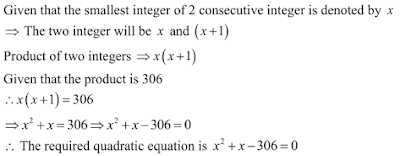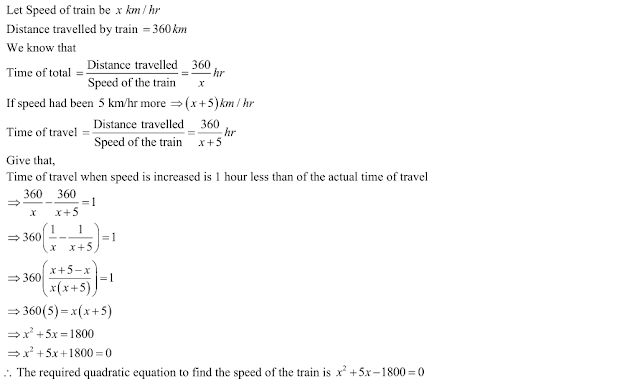## Chapter 8 Quadratic Equations R.D. Sharma Solutions for Class 10th Math Exercise 8.2

1. The product of two consecutive positive integers is 306. From the quadratic equation to find the integers , if x denotes the smaller integer.

Solution2. John and Jivanti together have 45 marbles. Both of them lost 5 marbles each , and the product of the number of marbles they now have is 128. Form the quadratic equation to find how many marbles they had to start with, if john had x marbles .

Solution3. A cottage industry produces a certain number of toys in a day. The cost of production of each toy (in rupees) was found to be 55 minus the number of articles produced in a day. On a particular day, the total cost of production was Rs. 750. If x denotes the number of toys produced that day, form the quadratic equation to find x.

Solution4. The height of a right triangle is 7 cm less than its base. If the hypotenuse is 13 cm, form the quadratic equation to find the base of the triangle.

Solution5. An express train takes 1 hour less than a passenger train to travel 132 km between Mysore and Bangalore. If the average speed of the express train is 1 1 km/hr more than that of the passenger train, form the quadratic equation to find the average speed of express train.

Solution6. A train travels 360 km at a uniform speed. If the speed had been 5 km/hr more, it would have taken 1 hour less for the same journey. Form the quadratic equation to find the speed of the train.

Solution